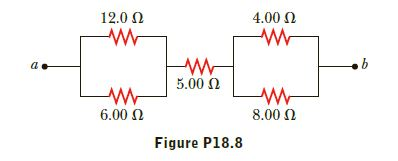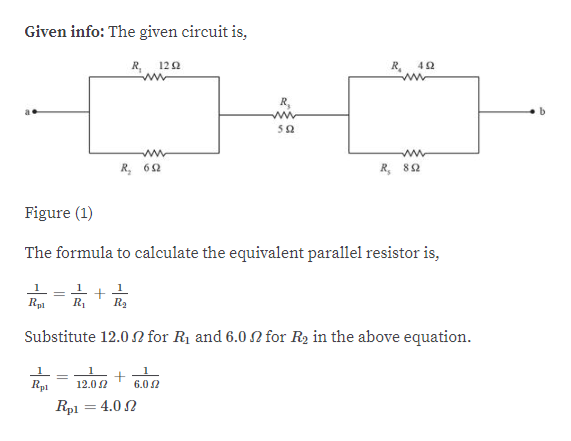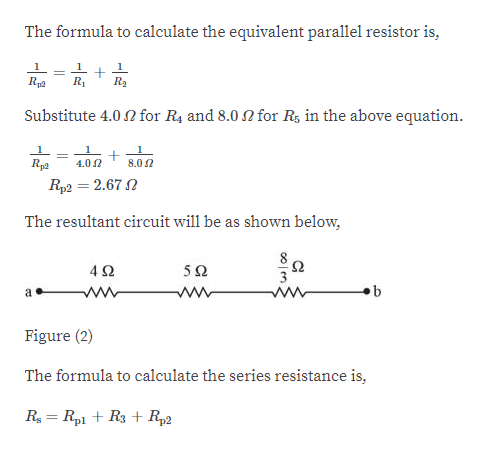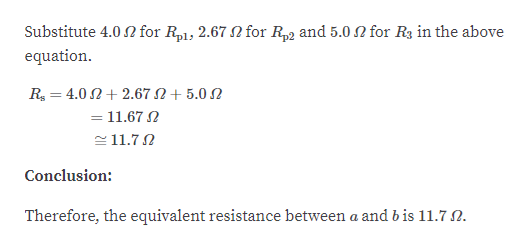Question
1 views

Consider the combination of resistors shown in Figure P18.8.
(a) Find the equivalent resistance between point a and b. (b)
If a voltage of 35.0 V is applied between points a and b, find
the current in each resistor.help_outlineImage Transcriptionclose12.0 N 4.00 N 5.00 N 8.00 N 6.00 N Figure P18.8 fullscreen
check_circle

Step 1help_outlineImage TranscriptioncloseGiven info: The given circuit is, R, 120 R, R, 50 R, 82 R, 62 Figure (1) The formula to calculate the equivalent parallel resistor is, Rp1 R1 R2 Substitute 12.0 N for R1 and 6.0 N for R2 in the above equation. 12.0 2 Rp1 6.0 2 Rp1 = 4.0 2 fullscreen
Step 2help_outlineImage TranscriptioncloseThe formula to calculate the equivalent parallel resistor is, Rp2 R1 R2 Substitute 4.0 N for R4 and 8.0 for R, in the above equation. Rp2 4.0 2 8.0 2 Rp2 = 2.67 N %3D The resultant circuit will be as shown below, 50 Figure (2) The formula to calculate the series resistance is, R = Rp1 + R3 + R22 fullscreen
Step 3help_outlineImage Transcriptionclose2 for R3 in the above Substitute 4.0 N for R1, 2.67 for R2 and 5.0 equation. R = 4.0 N+ 2.67 N+ 5.0 N = 11.67 N e 11.7N Conclusion: Therefore, the equivalent resistance between a and b is 11.7 N. fullscreen

### Want to see the full answer?

See Solution

#### Want to see this answer and more?

Solutions are written by subject experts who are available 24/7. Questions are typically answered within 1 hour.*

See Solution
*Response times may vary by subject and question.
Tagged in

### Current Electricity